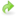# Expressing functionals¶

In the example presented in the tutorial, the quantity of interest was evaluated at the end of the simulation. However, it is very common to want to compute integrals over time, or evaluated at certain points in time that are not the end. With dolfin-adjoint this is very straightforward.

## Examples¶

To see how it works, we once again consider the Burgers equation example from the tutorial:

from fenics import *
from fenics_adjoint import *

n = 30
mesh = UnitSquareMesh(n, n)
V = VectorFunctionSpace(mesh, "CG", 2)

u = project(Expression(("sin(2*pi*x)", "cos(2*pi*x)"), degree=2),  V)
control = Control(u)

u_next = Function(V)
v = TestFunction(V)

nu = Constant(0.0001)

timestep = Constant(0.01)

F = (inner((u_next - u)/timestep, v)

bc = DirichletBC(V, (0.0, 0.0), "on_boundary")

t = 0.0
end = 0.1
while (t <= end):
solve(F == 0, u_next, bc)
u.assign(u_next)
t += float(timestep)

J = assemble(inner(u, u)*dx)
dJdu, dJdnu = compute_gradient(J, [control, Control(nu)])


Here the functional considered was

$J(u) = \int_{\Omega} \left\langle u(T), u(T) \right\rangle \ \textrm{d}\Omega.$

Let us see how we have to change the program to accomedate different functionals with different time dependencies: To do this we should change the forward code to compute part of $$J$$ at each time step and save the value to a list:

t = 0.0
end = 0.1
Jtemp = assemble(inner(u,u)*dx)
Jlist = [Jtemp]
while (t <= end):
solve(F == 0, u_next, bc)
u.assign(u_next)
t += float(timestep)

Jtemp = assemble(inner(u, u)*dx)
Jlist.append(Jtemp)


Let us look at some specific functionals:

• Integration over all time:

$J(u) = \int_0^T\int_{\Omega}\left\langle u(t),u(t)\right\rangle \ \textrm{d}\Omega \ \textrm{d}t$

We need to perform the time integral numerically, for example by the trapezoidal rule:

J = 0
for i in range(1, len(Jlist)):
J += 0.5*(Jlist[i-1] + Jlist[i])*float(timestep)


We could also use ready-made integration routines, but we have to make sure that the routine does not change the type of the J. Jtemp and J have type AdjFloat.Download the code to find the full time integral.

• Integration over a certain time window:

$J(u) = \int_{t_1}^{t_2}\int_{\Omega}\left\langle u(t),u(t)\right\rangle \ \textrm{d}\Omega \ \textrm{d}t$

We can again use the trapezoidal rule. Compared to the full time integration we only have to change the looping range. If we use our example with $$t_{1} = 0.03$$ and $$t_{2} = 0.07$$, then we can write

J = 0
for i in range(4, 8):
J += 0.5*(Jlist[i-1] + Jlist[i])*float(timestep)

• Integration from a certain point until the end:

$J(u) = \int_{t_1}^{T}\int_{\Omega}\left\langle u(t),u(t)\right\rangle \ \textrm{d}\Omega \ \textrm{d}t$

Again we only change the loop range. If we use our example with $$t_{1} = 0.03$$ we can write

J = 0
for i in range(4,len(Jlist)):
J += 0.5*(Jlist[i-1] + Jlist[i])*float(timestep)

• Pointwise evaluation in time:

$J(u) = \int_{\Omega}\left\langle u(t_1),u(t_1)\right\rangle \ \textrm{d}\Omega$

Here we only need to pick out the functional from the list, for example if $$t_1 = 0.03$$:

J = Jlist

• Pointwise evaluation at the start (e.g. for regularisation terms):

$J(u) = \int_{\Omega}\left\langle u(0),u(0)\right\rangle \ \textrm{d}\Omega$

Again we only need to pick out the functional from the list:

J = Jlist

• Pointwise evaluation at the end:

$J(u) = \int_{\Omega}\left\langle u(T),u(T)\right\rangle \ \textrm{d}\Omega$

Here we only need to pick out the functional from the list:

J = Jlist[-1]

• And sums of these work too:

$J(u) = \int_0^T\int_{\Omega}\left\langle u(t),u(t)\right\rangle \ \textrm{d}\Omega \ \textrm{d}t + \int_{\Omega}\left\langle u(T),u(T)\right\rangle \ \textrm{d}\Omega$
J = 0
for i in range(1, len(Jlist)):
J += 0.5*(Jlist[i-1] + Jlist[i])*float(timestep)
J += JList[-1]

• Ratio of evaluation at different times

$J(u) = \frac{\int_{\Omega}\left\langle u(t_2),u(t_2)\right\rangle \ \textrm{d}\Omega}{\int_{\Omega}\left\langle u(t_1),u(t_1)\right\rangle \ \textrm{d}\Omega}$

for example with $$t_1 = 0$$ and $$t_2 = 0.03$$:

J = Jlist*Jlist**(-1)


In the next section we discuss how to use pyadjoint with functions other than FEniCS functions.